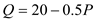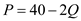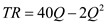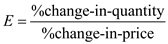# Quiz 6: Elasticity and Demand

Business

There are two different views about increasing the price of newspaper and its related increase in revenue: • The WSJ analysts explain that increasing price from 50 cents to 75 cents will bring in additional revenue of \$65 million. Their implicit assumption is that the elasticity of demand is inelastic. In other words an increase in price will have less effect on quantity demanded. And thus increase in price is greater than decrease in quantity, thus the overall Total revenue increases. However, publishers believe that the newspaper consumers are price sensitive. And an increase in price will not only decrease the quantity demanded to a large extent, but also decrease the total revenue heavily. This means they assume the elasticity of demand is elastic and thus consumers are price sensitive.

The given linear demand function equation is,Where , Q =quantity and p =price a. The inverse demand function is always expressed in terms of price:Thus, the inverse demand function is. b. Total revenue (TR) function is the product of quantity (Q) and price (P),Thus, the total revenue function is. c. The maximum point of any function can be calculated by differentiating the function and equating it to zero. The total revenue function's maximum can be calculated as follows:The maximizing quantity is 10. The price can be calculated by substituting the value of Q in the demand equation:Thus, the maximizing price is \$20. d. Marginal revenue function is derived by differentiating the total revenue function:The total revenue is maximized when the marginal revenue is equal to zero. Substituting the value of quantity equal to 10 in marginal revenue function, we get:Therefore, the total revenue is maximized at quantity 10. e. The elasticity (E) can be calculated at maximum price \$20 and MR =0 by using the below formula:The elasticity is unitary elastic. At maximizing point, the total revenue is maximum, the marginal revenue is zero and the elasticity is unit elastic.

Given that, plastic or cosmetic surgery is price inelastic, following interpretation would determine the correctness of each statement: a. With an inelastic price, the change in price will not affect the quantity demanded to great extent. Therefore a change in price will change the quantity demanded very little or not at all. However in normal goods price and quantity are inversely related. Therefore, the statement is true. b. The price elasticity of demand (E) can be calculated as follows:If the demand is inelastic, a change in price will cause a smaller change in quantity demanded. Therefore, the statement the change in price is less than change in quantity is false. c. If any product has elastic in demand, this means a change in price will cause a very small or no change in quantity demanded. The statement that, changes in price of plastic surgery does not affect the number of operations is true. d. The quantity demanded has an immediate effect by change in price if demand is elastic. With an inelastic demand, the quantity demanded is very unresponsive to price change. Thus, the statement is false. e. Expenditures on plastic demand will decrease due to economies of scale and production effectiveness and efficiency. The price elasticity has no role to play in cost efficiency. Hence, the statement is false. f. If there is inelasticity, an increase in quantity will decrease the total revenue. Therefore, the marginal revenue is negative with increase in quantity. Thus, the statement is true.